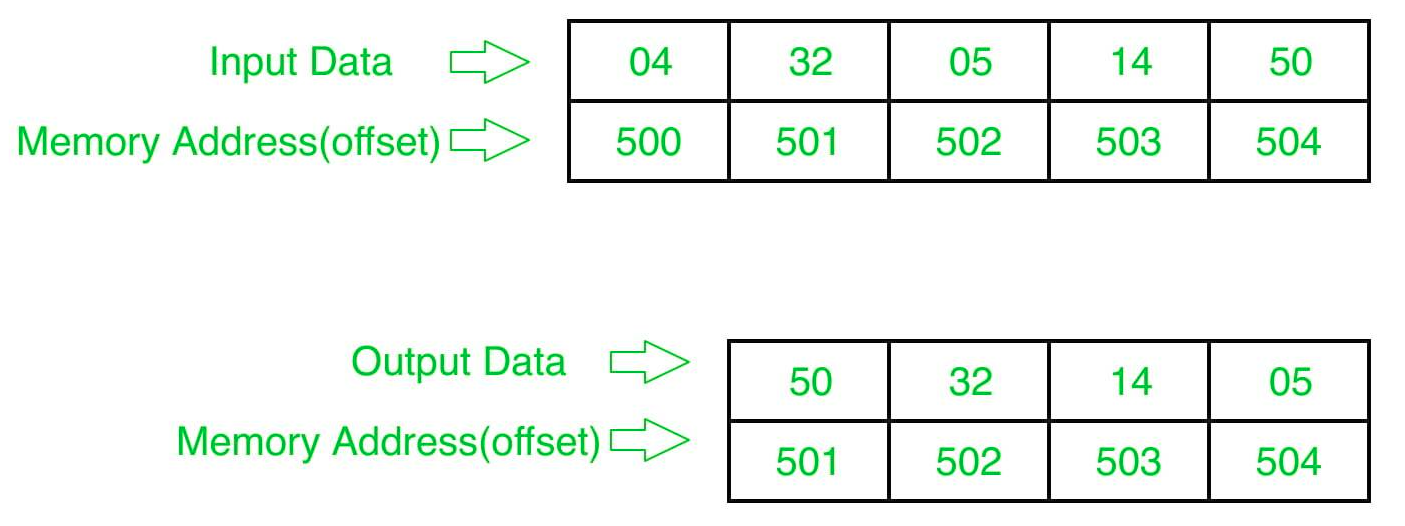# 8086 program to sort an integer array in descending order

• Last Updated : 29 Jun, 2018

Problem – Write a program in 8086 microprocessor to sort numbers in descending order in an array of n numbers, where size “n” is stored at memory address 2000 : 500 and the numbers are stored from memory address 2000 : 501.

Example –Example explanation:

```Pass-1:
32 05 14 50
32 05 14 50
32 14 05 50
32 14 50 05 (1 number got fix)

Pass-2:
32 14 50 05
32 14 50 05
32 50 14 05 (2 number got fix)

Pass-3:
32 50 14 05
50 32 14 05 (sorted)```

Algorithm –

1. Load data from offset 500 to register CL (for count).
2. Travel from starting memory location to last and compare two numbers if first number is smaller than second number then swap them.
3. First pass fix the position for last number.
4. Decrease the count by 1.
5. Again travel from starting memory location to (last-1, by help of count) and compare two numbers if first number is smaller than second number then swap them.
6. Second pass fix the position for last two numbers.
7. Repeate.

Program –

400MOV SI, 500SI<-500
403MOV CL, [SI]CL<-[SI]
405DEC CLCL<-CL-1
407MOV SI, 500SI<-500
40AMOV CH, [SI]CH<-[SI]
40CDEC CHCH<-CH-1
40EINC SISI<-SI+1
40FMOV AL, [SI]AL<-[SI]
411INC SISI<-SI+1
412CMP AL, [SI]AL-[SI]
416XCHG AL, [SI]SWAP AL AND [SI]
418DEC SISI<-SI-1
419XCHG AL, [SI]SWAP AL AND [SI]
41BINC SISI<-SI+1
41CDEC CHCH<-CH-1
420DEC CLCL<-CL-1
424HLTEND

Explanation –

1. MOV SI, 500: set the value of SI to 500.
2. MOV CL, [SI]: load data from offset SI to register CL.
3. DEC CL: decrease value of register CL BY 1.
4. MOV SI, 500: set the value of SI to 500.
5. MOV CH, [SI]: load data from offset SI to register CH.
6. DEC CH: decrease value of register CH BY 1.
7. INC SI: increase value of SI BY 1.
8. MOV AL, [SI]: load value from offset SI to register AL.
9. INC SI: increase value of SI BY 1.
10. CMP AL, [SI]: compares value of register AL and [SI] (AL-[SI]).